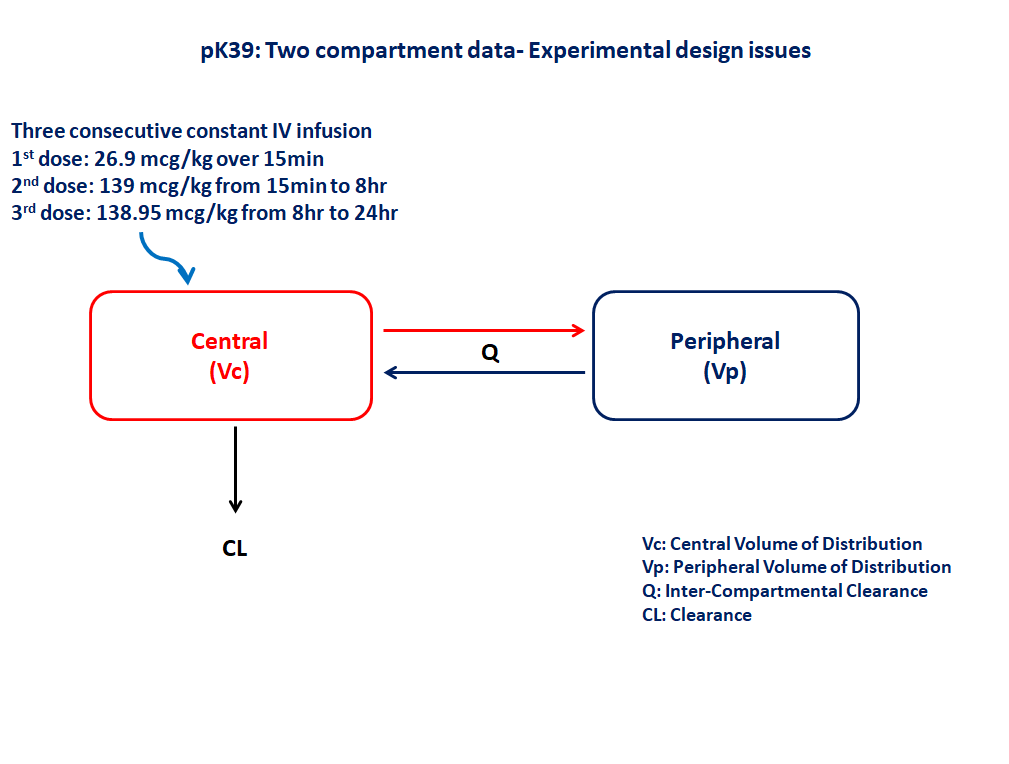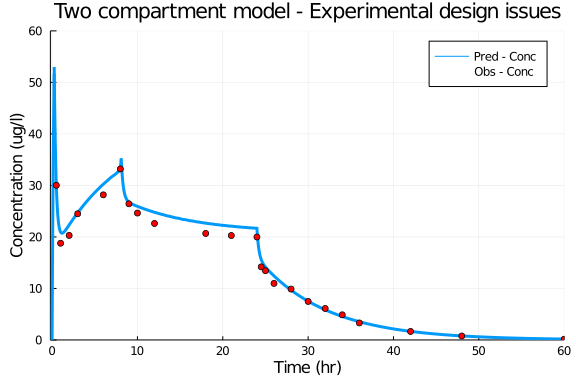# Exercise PK39 - Two Compartment data-Experimental design issues

### Background

• Structural model - Two compartment linear elimination with zero order absorption

• Route of administration - Three consecutive constant rate IV infusion

• Dosage Regimen - 1st dose:- 26.9 mcg/kg over 15min, 2nd dose:- 139 mcg/kg from 15min to 8hr, 3rd dose:- 138.95 mcg/kg between 8hr to 24hr

• Number of Subjects - 1### Learning Outcome

In this model, "Two compartment model- Experimental design" issues helps in understanding how to fit a model to the observed data and further to assess the impact of the best experimental design for estimation.

### Objectives

In this model you will learn how to build a two compartment model and simulate for a single subject.

### Libraries

call the "necessary" libraries to get start.

using Pumas
using Plots
using CSV
using StatsPlots
using Random


### Model

In this two compartment model we administer three consecutive IV infusion for a single subject and we assess the disposition of drug and fitting the model to the observed data.

pk_39           = @model begin
@param begin
tvcl        ∈ RealDomain(lower=0)
tvvc        ∈ RealDomain(lower=0)
tvvp        ∈ RealDomain(lower=0)
tvq         ∈ RealDomain(lower=0)
Ω           ∈ PDiagDomain(4)
σ²_prop     ∈ RealDomain(lower=0)
end

@random begin
η           ~ MvNormal(Ω)
end

@pre begin
CL          = tvcl * exp(η)
Vc          = tvvc * exp(η)
Vp          = tvvp * exp(η)
Q           = tvq * exp(η)
end

@dynamics begin
Central'    =  (Q/Vp)*Peripheral - (Q/Vc)*Central -(CL/Vc)*Central
Peripheral' = -(Q/Vp)*Peripheral + (Q/Vc)*Central
end

@derived begin
cp          = @. Central/Vc
dv          ~ @. Normal(cp, sqrt(cp^2*σ²_prop))
end
end

PumasModel
Parameters: tvcl, tvvc, tvvp, tvq, Ω, σ²_prop
Random effects: η
Covariates:
Dynamical variables: Central, Peripheral
Derived: cp, dv
Observed: cp, dv


### Parameters

Parameters provided for simulation. tv represents the typical value for parameters.

• Cl - Clearance (L/kg/hr)

• Vc - Volume of Central Compartment (L/kg)

• Vp - Volume of Peripheral Compartment (L/kg)

• Q - Intercompartmental clearance (L/kg/hr)

• Ω - Between Subject Variability

• σ - Residual error

param = (tvcl    = 0.417793,
tvvc    = 0.320672,
tvvp    = 2.12265,
tvq     = 0.903188,
Ω       = Diagonal([0.0,0.0,0.0,0.0]),
σ²_prop = 0.005)

(tvcl = 0.417793, tvvc = 0.320672, tvvp = 2.12265, tvq = 0.903188, Ω = [0.0
0.0 0.0 0.0; 0.0 0.0 0.0 0.0; 0.0 0.0 0.0 0.0; 0.0 0.0 0.0 0.0], σ²_prop =
0.005)


### Dosage Regimen

Single subject receiving three consecutive IV infusion

• 1st dose: 26.9 mcg/kg over 15min

• 2nd dose: 139 mcg/kg from 15min to 8hr

• 3rd dose: 138.95 mcg/kg between 8hr to 24hr

ev1  = DosageRegimen(26.9, time=0, cmt=1, duration=0.25)
ev2  = DosageRegimen(139, time=0.25, cmt=1, duration=7.85)
ev3  = DosageRegimen(138.95, time=8, cmt=1, duration=16)
evs  = DosageRegimen(ev1,ev2,ev3)
sub1 = Subject(id=1, events=evs)

Subject
ID: 1
Events: 6


### Simulation

Lets simulate for plasma concentration with the specific observation time points after IV infusion.

Random.seed!(123)
sim_sub1 = simobs(pk_39, sub1, param, obstimes=0:0.01:60)
df1      = DataFrame(sim_sub1)


### Dataframe and plots

Save the simulated data into a dataframe to plots

df1_dv = filter(x -> x.time in [0.25,0.5,1,2,3,6,8,9,10,12,18,21,24,24.5,25,26,28,30,32,34,36,42,48,60], df1)

@df df1 plot(:time, :cp,
title="Two compartment model - Experimental design issues",label="Pred - Conc",
xlabel="Time (hr)",ylabel="Concentration (ug/l)", linewidth=3,
xticks=[0,10,20,30,40,50,60], xlims=(-0.2,60), yticks=[0,10,20,30,40,50,60], ylims=(0,60))
@df df1_dv scatter!(:time,:dv, label="Obs - Conc", color=[:red])### Additional notes

Experimental design in this exercise deals with reducing the 24 observation dataset to 14 observation and 5 observation and fitting the two compartment model to these datasets to estimate final parameters.